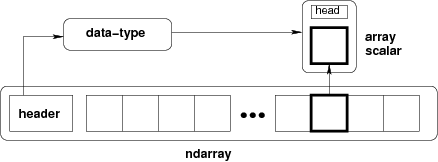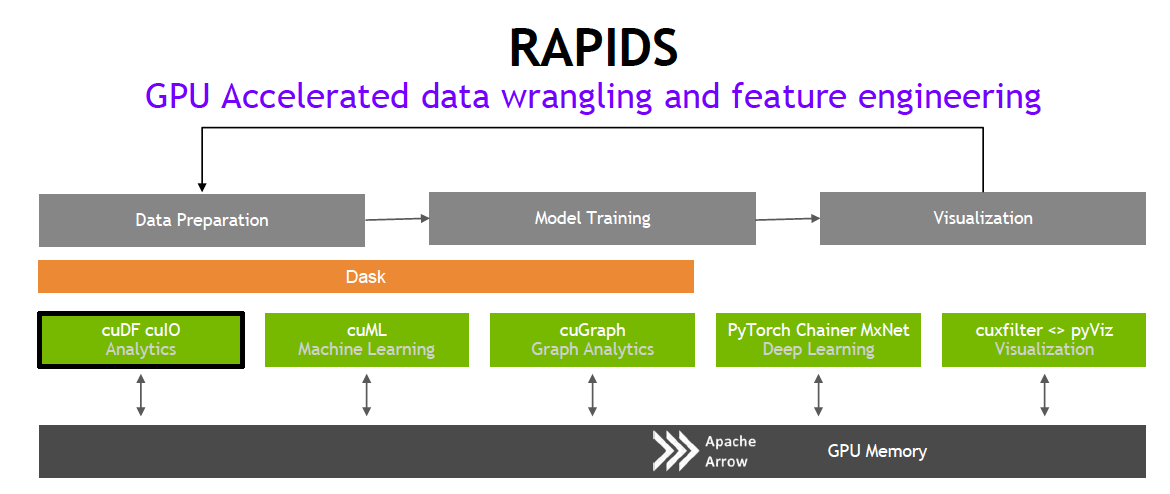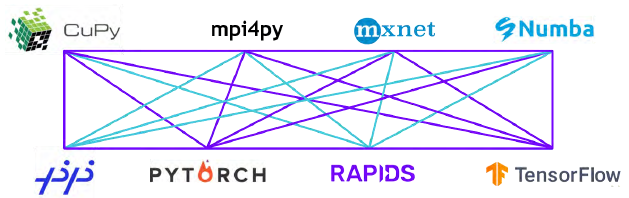# NumPy

NumPy is a free, open-source Python library for n-dimensional array (also known as tensors) processing and numerical computing.

## What is NumPy

NumPy is a powerful, well-optimized, free open-source library for the Python programming language, adding support for large, multi-dimensional arrays (also called matrices or tensors). NumPy also comes equipped with a collection of high-level mathematical functions to work in conjunction with these arrays. These include basic linear algebra, random simulation, Fourier transforms, trigonometric operations, and statistical operations.

NumPy stands for ‘numerical Python’, and builds on the early work of the Numeric and Numarray libraries with the goal to give fast numeric computation to Python.  Today NumPy has numerous contributors and is sponsored by NumFOCUS.

As the core library for scientific computing, NumPy is the base for libraries such as Pandas, Scikit-learn, and SciPy. It’s widely used for performing optimized mathematical operations on large arrays.

## Why NumPy—and How it Works

A multidimensional array is a central data structure of a NumPy library, and generically represents a grid of values. NumPy’s ndarray, a homogeneous n-dimensional array object, describes a collection of elements or items of a similar type. Within these ndarrays, each item comprises the same size memory block and each block is identified the same way. This enables efficient, fast, and easy manipulation of data for scientific computing.NumPy array operations are faster than Python Lists because NumPy arrays are compilations of similar data types and are packed densely in memory. By contrast, a Python List can have varying data types, placing additional constraints on the system while performing computation upon them.

Benefits of NumPy

Important benefits and properties of NumPy include:

• NumPy’s ndarray computing concepts are at the core of the scientific Python and PyData ecosystems.
• NumPy provides a Python front-end for highly optimized C functions allowing for a simple Python interface with the speed of compiled code.
• NumPy’s powerful N-dimensional array object integrates with a wide variety of libraries.
• NumPy arrays can execute advanced mathematical operations with large data sets more efficiently and with less code than when using Python’s built-in lists. This is critical for scientific computing sequence, where size and speed are vital.

## Why NumPy Matters to You

NumPy gives data scientists the blend of Python usability and C-level optimization needed to enable quick realization of efficient code for exploratory data analysis, as well as model building. This blend is imperative for the rapid prototyping of algorithms necessary to be successful in scientific computing today. This has made NumPy the de facto way of communicating multi-dimensional data in Python.

## GPU-Accelerated Computing with Python

Architecturally, the CPU is composed of just a few cores with lots of cache memory that can handle a few software threads at a time. In contrast, a GPU is composed of hundreds of cores that can handle thousands of threads simultaneously.NumPy has become the de facto way of communicating multi-dimensional data in Python. However, its implementation is not optimal for many-core GPUs.  For this reason, newer libraries optimized for GPUs implement or interoperate with the Numpy array.

NVIDIA® CUDA® is a parallel computing platform and programming model developed by NVIDIA for general computing on GPUs. The CUDA array interface is a standard format that describes a GPU array (tensor) to allow sharing GPU arrays between different libraries without needing to copy or convert data. CUDA array is supported by Numba, CuPy, MXNet, and PyTorch.

• CuPy is a library that implements NumPy arrays on NVIDIA GPUs by leveraging the CUDA GPU library.
• Numba is a Python compiler that can compile Python code for execution on CUDA-capable GPUs. NumPy arrays are directly supported in Numba.
• Apache MXNet is a flexible and efficient library for deep learning. Its NDArray is used to represent and manipulate the inputs and outputs of a model as multi-dimensional arrays. NDArray is similar to NumPy’s ndarrays, but they can run on GPUs to accelerate computing.
• PyTorch is an open-source deep learning framework that’s known for its flexibility and ease-of-use. Pytorch Tensors are similar to NumPy’s ndarrays, except they can run on GPUs to accelerate computing.

## NVIDIA GPU-Accelerated, End-to-End Data Science

The NVIDIA RAPIDS suite of open-source software libraries, built on CUDA, provides the ability to execute end-to-end data science and analytics pipelines entirely on GPUs. It relies on NVIDIA CUDA primitives for low-level compute optimization, but exposes that GPU parallelism and high-bandwidth memory speed through user-friendly Python interfaces.

With the RAPIDS GPU DataFrame, data can be loaded onto GPUs using a Pandas-like interface, and then used for various connected machine learning and graph analytics algorithms without ever leaving the GPU. This level of interoperability is made possible through libraries like Apache Arrow. You can create a GPU dataframe from NumPy arrays, Pandas DataFrames, and PyArrow tables with just a single line of code. Other projects can exchange data using the CUDA array interface. This allows acceleration for end-to-end pipelines—from data prep to machine learning to deep learning.RAPIDS supports device memory sharing between many popular data science libraries. This keeps data on the GPU and avoids costly copying back and forth to host memory.